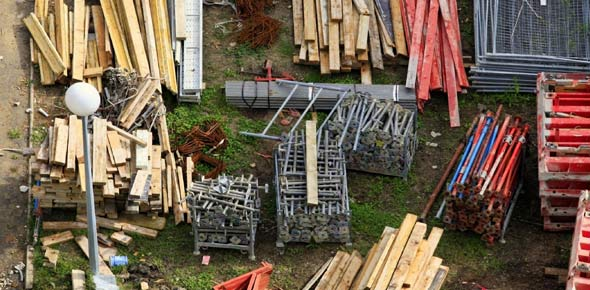# Strength Of Materials! Trivia Questions Quiz

12 Questions | Total Attempts: 324SettingsThis is a strength of materials trivia questions quiz. When it comes to fields such as engineering and construction it is important for one to understand the changes that different materials face when they go through certain processes. If you are looking to test how well you know different materials and how they change based on force applied, this quiz is for you. Give it a shot and see what more you may learn.

• 1.
In a compression on mild steel
• A.

Necking does not occur

• B.

Hooke's law is not valid

• C.

Hooke's law is valid beyond yield point

• D.

Strength in compression is much greater than the strength in tension

• 2.
For most brittle materials generally ultimate strength in compression is much larger than the ultimate strength in tension, because
• A.

Of flaws such as microscopic cracks or cavities

• B.

Compression failure is due to normal stress, and failure in tension due to shear stress

• C.

Yield point does not occur in compression

• D.

Of inherent properties of materials

• 3.
Which of the following statement is false?
• A.

The stress dependent part of the plastic deformation is referred to as creep, and the time dependent part which is also influenced by the temperature as slip

• B.

In case of ductile material possessing a well-defined yield point, the proportional limit almost coincides

• C.

A fatigue failure is of a brittle nature even for materials which are normally ductile

• D.

The endurance limit for machined and polished specimens is higher than for rolled or forged components

• 4.
For carbon steel, the endurance limit is approximately
• A.

0.3

• B.

0.5

• C.

0.75

• D.

0.85

• 5.
The yield stress of a twisted bar as compared to an ordinary mild steel bar is nearly
• A.

50% more

• B.

25% more

• C.

50% less

• D.

25% less

• 6.
Which of the following is primarily responsible for toughness of steel?
• A.

Iron

• B.

Carbon

• C.

Manganese

• D.

Phosphorous

• 7.
As compared to mild steel, high carbon steel has less
• A.

Ultimate strength

• B.

Hardness

• C.

Ductility

• D.

Hardness and ductility

• 8.
Consider the following statements: A: An isotropic material is always homogeneous R: An isotropic material is one in which all the properties are the same in all the directions at every point. Of these statements
• A.

Both A and R are true and R is the correct explanation of A

• B.

Both A and R are true but R is not a correct explanation of A

• C.

A is true but R is false

• D.

A is false but R is true

• 9.
Consider the following statements regarding tensile test diagrams for carbon steels with varying carbon contents. As the carbon content increases 1. the ultimate strength of steel decreases 2. the elongation before fracture increases 3. the ductility of the metal decreases 4. the percentage elongation of steel decreases Of these statements
• A.

3 and 4 are correct

• B.

1 and 3 are correct

• C.

1, 2 and 3 are correct

• D.

1 and 2 are correct

• 10.
Consider the following factors: 1. Large number of loading cycles 2. Large variations in stress 3. Large stress concentrations Those associated with fatigue failure include
• A.

1 and 2

• B.

1 and 3

• C.

2 and 3

• D.

1, 2 and 3

• 11.
A deviator state of stress is characterized by
• A.

A change in volume without distortion

• B.

A distortion without change in volume

• C.

A change in volume accompanied by distortion

• D.

No change in either volume nor distortion

• 12.
Which of the following methods of design would be suitable for metal structures subjected to stress reversals and impact? 1. Simple working stress design 2. Rigid plastic design 3. Semi-rigid design 4. Elastic rigid design Select the correct answer using the codes given below:
• A.

1, 2 and 4

• B.

1, 3 and 4

• C.

1, 2 and 3

• D.

2, 3 and 4

Related TopicsBack to top